+关注继续查看

# 多变量赋值

--多变量赋值
a,b,c='123',666,true
print(a)
print(b)
print(c)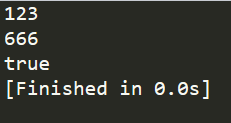--全自动赋值
--变量少了，自动补空
t1,t2,t3=1,2
print(t1)
print(t2)
print(t3)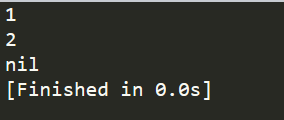--变量多了，自动裁剪
t1,t2,t3=1,2,6,7,8
print(t1)
print(t2)
print(t3)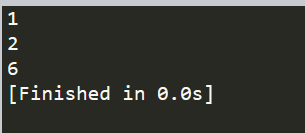# 函数多返回值

--多返回值赋值
function Test()
return 1,2,3,4
end

--获得返回值也是一样的，全自动的，多退少补
a,b,c=Test()
print(a)
print(b)
print(c)
print('-------------------------------')
a,b,c,d,e,f=Test()
print(a)
print(b)
print(c)
print(d)
print(e)
print(f)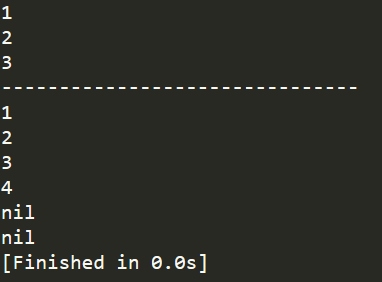# and or(在lua内的逻辑运算符)

and or 不仅可以与bool 其他任何东西也可以连


--lua中 只有 nil\false 才认为是假
--'短路'   对于and来说 有假则假   or来说 有真则真
--因此 只需要判断第一个是否满足 就会停止判断
print(1 and 2) --因为只有 nil\false 才认为是假，所以程序会继续执行到2
print(0 and 1) --同上
print(nil and 1) --因为只有 nil\false 才认为是假，所以程序停止执行下一个，返回nil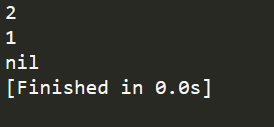--or测试
--遇真则真，遇到真就不会再去计算后面的值了
print(1 or 2)
print(0 or 1)
print(nil or 1)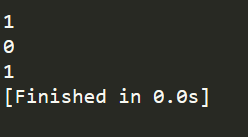--lua虽然不支持三目运算符
--但我们可用lua 逻辑运算符实现
x=3
y=2
res = (x>y) and x or y
print(res)
--(x>y) and x -> x    and遇假则假
--x or y -> x         or遇真则真

x=1
res = (x>y) and x or y
print(res)
--（x>y）and x ->(x>y)
-- (x>y) or y -> y   (x>y)返回的是false(假) ， or遇真则真，所以返回y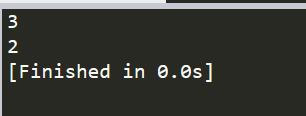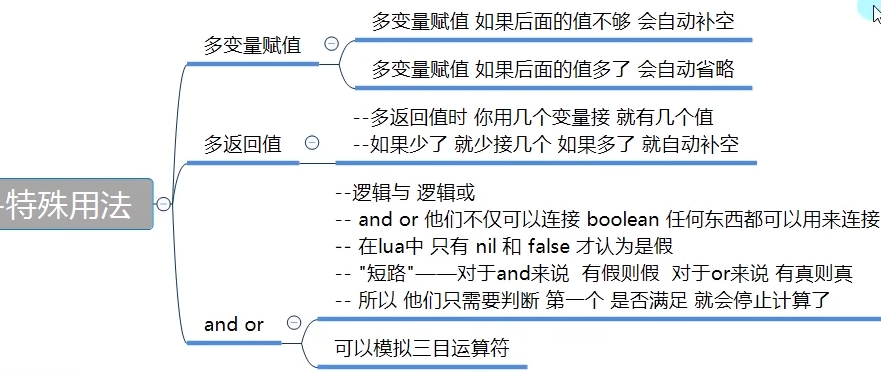|
1月前
|

lua变量、数据类型、if判断条件和数据结构table以及【lua 函数】
lua变量、数据类型、if判断条件和数据结构table以及【lua 函数】
22 0
|
7月前
[✔️]lua中的module函数
[✔️]lua中的module函数
80 0
|
12月前
|
C语言 索引
lua语言——函数
lua语言——函数
91 0
lua 日期的一些函数
--根据日期获取星期几 function getWeekNum(strDate) local ymd = Split(strDate,"-") t = os.time({year=tostring(ymd),month=tostring(ymd),day=tostring(ymd)}) local weekNum = os.
1581 0